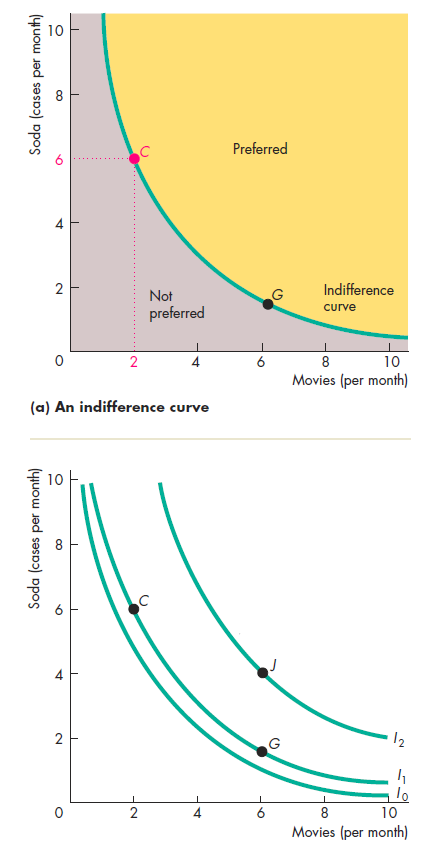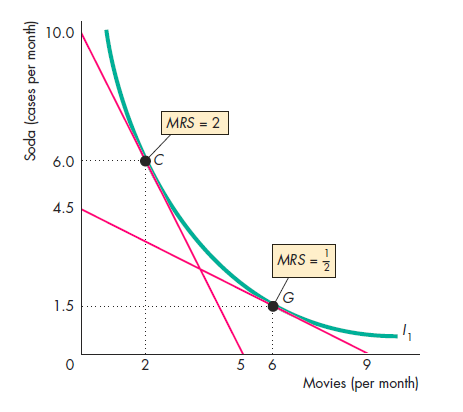# Central London Economics Tutors hired from LSE, Kings, UCL.#### Indifference Curves:

An indifference curve is an iso-utility curve that represents all points that have the same utility. It means that a consumer should be indifferent at any point on the curve because all points represent the same level of utility.

##Loading...Taking too long?Reload document |Open in new tab

#### Axioms of Indifference Curves:

1. Monotonicity means more is always preferred to less. For example, five bananas will always be preferred to four bananas. Utility of (x+1) will always be higher than U(x).
2. Continuity means that bundles can be broken down into infinitely small bundles. The idea is to have indifference curves that are continuous and not discrete points of certain (whole) bundles.
3. Convexity means that consumers prefer average bundles to extreme bundles. Suppose a consumer is indifference between two bundles A and B such that U(A) = U(B). If we introduce a bundle C which is the average of A and B such that C = (A+B)/2 then U(C) > U(A) ~ U(B). This means that the consumer gets more utility from the average bundle than the extreme bundles.

#### Marginal rate of substitutionThe marginal rate of substitution represents the rate at which a consumer gives up good y for a unit of good x in order to stay indifferent (have the same utility). The MRS for a convex function is not constant. In fact, it is diminishing. Different preferences have different types of MRS. Work with Central London Economics Tutors for A levels, IB Economics in and around London. We hire economics tutors from LSE, Kings, Warwick, UCL, Imperial, SOAS.

##Loading...Taking too long?Reload document |Open in new tab

Microeconomics Lesson Calculus Review

London Economics Tutor Utililty Functions

Other popular courses:

Get Started

## See the #1 economics mentoring platform in action

Request a demo